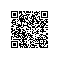# jQuery 选择器

#id $("#lastname") id="lastname" 的元素 .class$(".intro") 所有 class="intro" 的元素
element $("p") 所有 <p> 元素 .class.class$(".intro.demo") 所有 class="intro" 且 class="demo" 的元素

:first $("p:first") 第一个 <p> 元素 :last$("p:last") 最后一个 <p> 元素
:even $("tr:even") 所有偶数 <tr> 元素 :odd$("tr:odd") 所有奇数 <tr> 元素

:eq(index) $("ul li:eq(3)") 列表中的第四个元素（index 从 0 开始） :gt(no)$("ul li:gt(3)") 列出 index 大于 3 的元素
:lt(no) $("ul li:lt(3)") 列出 index 小于 3 的元素 :not(selector)$("input:not(:empty)") 所有不为空的 input 元素

:header $(":header") 所有标题元素 <h1> - <h6> :animated 所有动画元素 :contains(text)$(":contains('W3School')") 包含指定字符串的所有元素
:empty $(":empty") 无子（元素）节点的所有元素 :hidden$("p:hidden") 所有隐藏的 <p> 元素
:visible $("table:visible") 所有可见的表格 s1,s2,s3$("th,td,.intro") 所有带有匹配选择的元素

[attribute] $("[href]") 所有带有 href 属性的元素 [attribute=value]$("[href='#']") 所有 href 属性的值等于 "#" 的元素
[attribute!=value] $("[href!='#']") 所有 href 属性的值不等于 "#" 的元素 [attribute$=value] $("[href$='.jpg']") 所有 href 属性的值包含以 ".jpg" 结尾的元素

:input $(":input") 所有 <input> 元素 :text$(":text") 所有 type="text" 的 <input> 元素
:password $(":password") 所有 type="password" 的 <input> 元素 :radio$(":radio") 所有 type="radio" 的 <input> 元素
:checkbox $(":checkbox") 所有 type="checkbox" 的 <input> 元素 :submit$(":submit") 所有 type="submit" 的 <input> 元素
:reset $(":reset") 所有 type="reset" 的 <input> 元素 :button$(":button") 所有 type="button" 的 <input> 元素
:image $(":image") 所有 type="image" 的 <input> 元素 :file$(":file") 所有 type="file" 的 <input> 元素

:enabled $(":enabled") 所有激活的 input 元素 :disabled$(":disabled") 所有禁用的 input 元素
:selected $(":selected") 所有被选取的 input 元素 :checked$(":checked") 所有被选中的 input 元素使用钉钉扫一扫加入圈子
+ 订阅